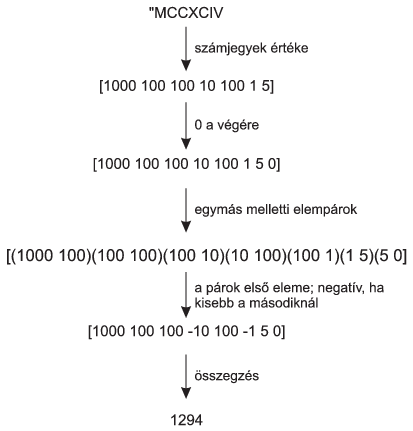Mathematical and Physical Journal
for High Schools
Issued by the MATFUND Foundation
 Already signed up? New to KöMaL?

#Problem I. 387. (November 2015)

I. 387. Converting Roman numerals to Arabic numbers was the topic of the spreadsheet management task of the practical part of the advanced level Matriculation Examination in Informatics in Hungary in May 2012. The algorithm given there can also be realized by using functional programming.

By using a Logo program and the given algorithm, convert Roman numerals to Arabic numbers. You can assume that the input Roman numerals (between 1 and 4000, and described by at most 20 capital letters) are syntactically correct.

The conversion algorithm is described below.Here a számjegyek értéke'' is the values of the numerals''; 0 a végére'' is appending 0 at the end''; az egymás melletti elempárok'' is adjacent pairs''; a párok első eleme'' is the first entries of the pairs''; negatív, ha kisebb a másodiknál'' is negative if the first entry is smaller than the second one''; and összegzés" is summation''.

The sign of a given numeral is negative if the following numeral is larger. The value of the last numeral is always positive.

Create the Logo words corresponding to the above steps of the algorithm, then the word római_tízes performing the actual conversion.

 Sample command Result római_tízes "MCCXCIV 1294

Only the automatic and functional features of the programming language should be used in your solution; variables should not be used just parameterizations.

The project file/source code of your program should be submitted (i387.imp).

(10 pont)

Deadline expired on December 10, 2015.

### Statistics:

 5 students sent a solution. 10 points: Kovács 246 Benedek, Nagy Ábel, Olexó Gergely, Radnai Bálint, Uzonyi 000 Ákos.

Problems in Information Technology of KöMaL, November 2015